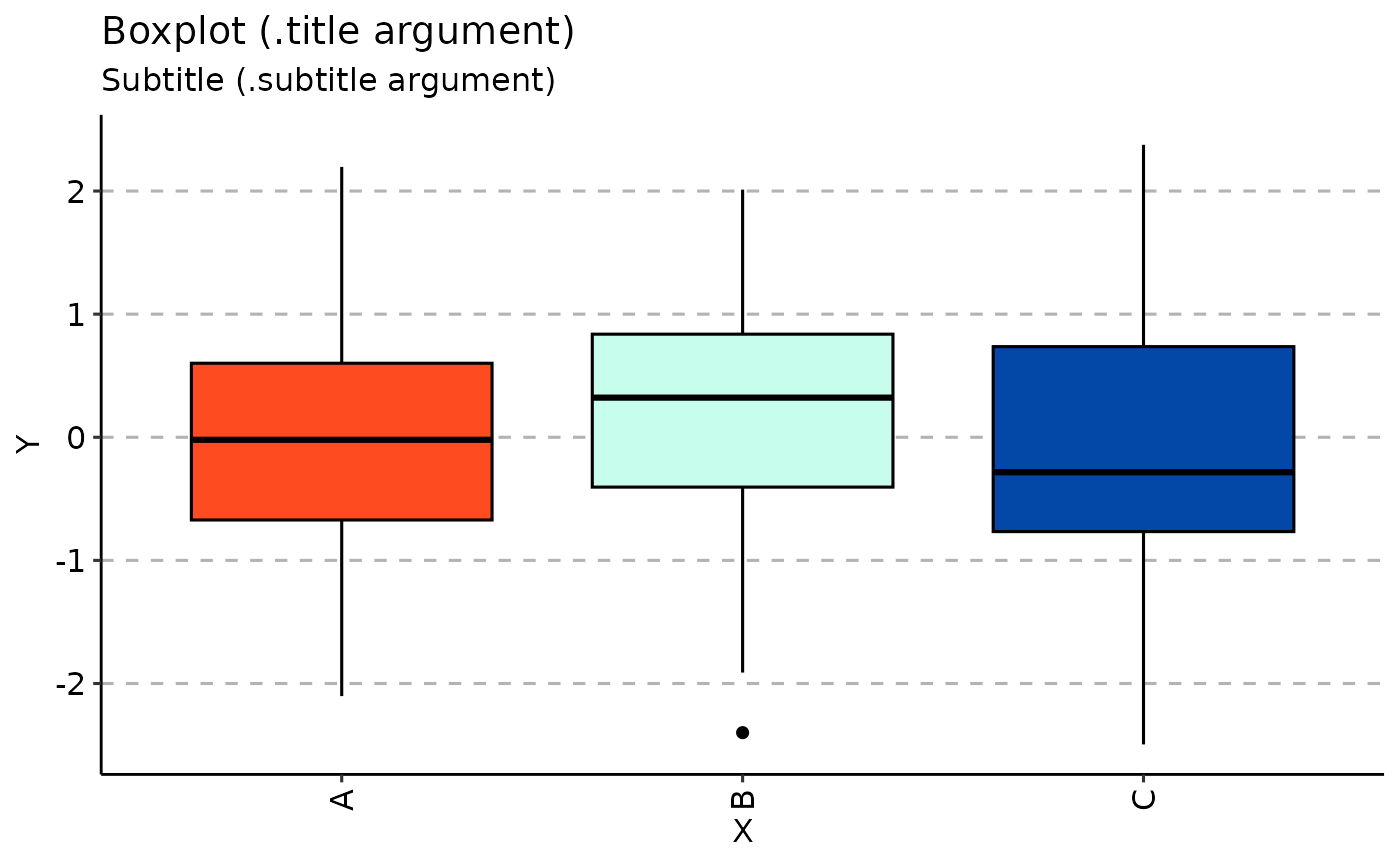Visualisation of distributions using ggplot2-based boxplots.

vis_box(
.data,
.by = NA,
.meta = NA,
.melt = TRUE,
.points = TRUE,
.test = TRUE,
.signif.label.size = 3.5,
.defgroupby = "Sample",
.grouping.var = "Group",
.labs = c("X", "Y"),
.title = "Boxplot (.title argument)",
.subtitle = "Subtitle (.subtitle argument)",
.legend = NA,
.leg.title = "Legend (.leg.title argument)",
.legend.pos = "right"
)

## Arguments

.data Input matrix or data frame. Pass NA if you want to plot samples without grouping. You can pass a character vector with one or several column names from ".meta" to group your data before plotting. In this case you should provide ".meta". You can pass a character vector that exactly matches the number of samples in your data, each value should correspond to a sample's property. It will be used to group data based on the values provided. Note that in this case you should pass NA to ".meta". A metadata object. An R dataframe with sample names and their properties, such as age, serostatus or hla. If TRUE then apply melt to the ".data" before plotting. In this case ".data" is supposed to be a data frame with the first character column reserved for names of genes and other numeric columns reserved to counts or frequencies of genes. Each numeric column should be associated with a specific repertoire sample. A logical value defining whether points will be visualised or not. A logical vector whether statistical tests should be applied. See "Details" for more information. An integer value defining the size of text for p-value. A name for the column with sample names. A name for the column to group by. Character vector of length two with names for x-axis and y-axis, respectively. The text for the title of the plot. The The text for the plot's subtitle. If TRUE then displays a legend, otherwise removes legend from the plot. The The text for the plots's legend. Provide NULL to remove the legend's title completely. Positions of the legend: either "top", "bottom", "left" or "right".

## Value

A ggplot2 object.

vis_box(data.frame(Sample = sample(c("A", "B", "C"), 100, TRUE), Value = rnorm(100)), .melt = FALSE)